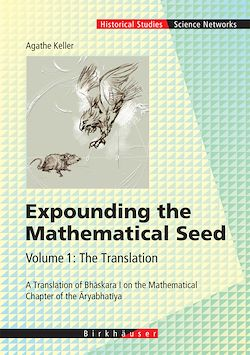172 Pages
English

# Expounding the Mathematical Seed. Vol. 1: The Translation

-Description

In the 5th century the Indian mathematician Aryabhata (476-499) wrote a small but famous work on astronomy, the Aryabhatiya. This treatise, written in 118 verses, gives in its second chapter a summary of Hindu mathematics up to that time. Two hundred years later, an Indian astronomer called Bhaskara glossed this mathematial chapter of the Aryabhatiya.

An english translation of Bhaskara’s commentary and a mathematical supplement are presented in two volumes.

Subjects treated in Bhaskara’s commentary range from computing the volume of an equilateral tetrahedron to the interest on a loaned capital, from computations on series to an elaborate process to solve a Diophantine equation.

This volume contains an introduction and the literal translation.

The introduction aims at providing a general background for the translation and is divided in three sections: the first locates Bhaskara’s text, the second looks at its mathematical contents and the third section analyzes the relations of the commentary and the treatise.

Subjects

##### Astronomy

Informations